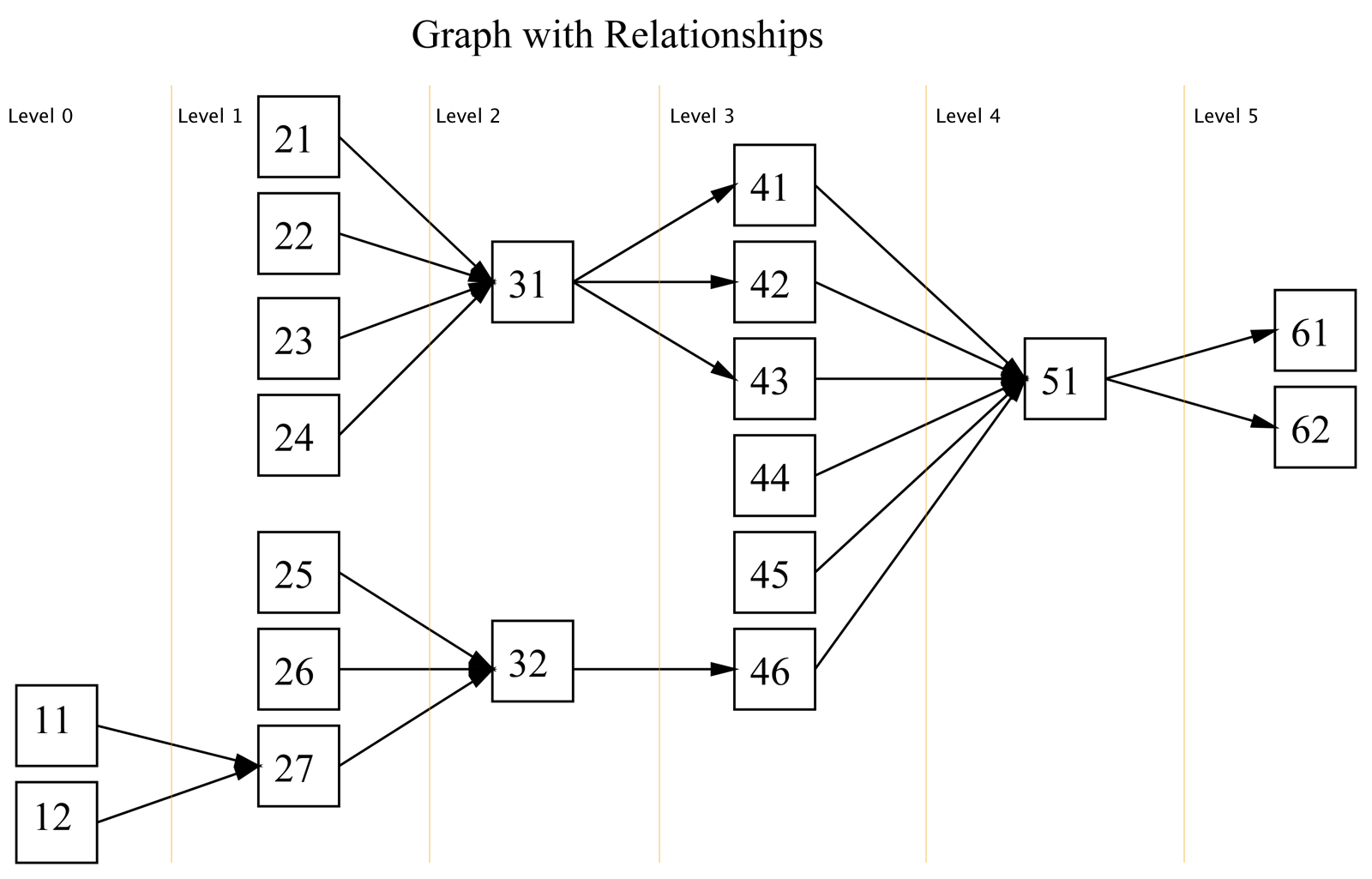# Orc.DependencyGraph

## Introduction

This library will help navigate a directed acyclic graph (DAG).

The goal of this library is to make it easy to:

• Find a specific node within a graph.
• Find all nodes on a certain level of the graph.
• Find all nodes between two levels of the graph.
• Find all nodes related to a given node. (i.e. find its decedents and/or its precedents on any level of the graph.)
• Sort the nodes in topological order

## Naming Convention

• Descendants. i.e. What descends from or comes after: Child
• Precedents. i.e What precedes, or comes before: Parent
• Level. We consider level as topological level of the node. I.e. Level 1 consists of nodes whose Precedents are of Level 0. In general level is the longest path from the node to the root of the graph.

## Interface

### Graph

``````public interface IGraph<T>
where T : IEquatable<T>
{
INode<T> Find(T value);

IEnumerable<INode<T>> Nodes { get; }

bool CanSort();
bool CanSort(IEnumerable<T> sequence);

int CountNodes { get; }
int CountLevels { get; }
IOrderedEnumerable<INode<T>> GetNodes(int level);
IOrderedEnumerable<INode<T>> GetNodesBetween(int levelFrom, int levelTo);
IOrderedEnumerable<INode<T>> Sort();
}
``````

Note:

• `AddSequence(IEnumerable<T> sequence)`: the sequence must contain at least 2 items. The relationship between the items is automatically assumed as item1 -> item2 -> item3 etc…

### Node

``````public interface INode<T>
where T: IEquatable<T>
{
T Value { get; }
IGraph<T> Graph { get; }
int Level { get; }

// relativeLevel >= relativeLevelFrom && relativeLevel <= relativeLevelTo
IOrderedEnumerable<INode<T>> GetNeighbours(int relativeLevelFrom, int relativeLevelTo);
// relativeLevel < 0
IOrderedEnumerable<INode<T>> Precedents { get; }
// relativeLevel > 0
IOrderedEnumerable<INode<T>> Descendants { get; }
// relativeLevel == relativeLevel - 1
IOrderedEnumerable<INode<T>> ImmediatePrecedents { get; }
// relativeLevel == relativeLevel + 1
IOrderedEnumerable<INode<T>> ImmediateDescendants { get; }
// Precedents of the node without precedents (roots)
IOrderedEnumerable<INode<T>> TerminatingPrecedents { get; }
// Descendants of the node without descendants (leafs)
IOrderedEnumerable<INode<T>> TerminatingDescendants { get; }
}
``````

Note:

• All the methods return an ordered enumerable of INode. The ordering is based on the “level” of the node. (Within a level the ordering is not important.)
• If possible the methods returns all the INodes lazily.
• A Node object has a reference to the Graph object.

## Algorithms, Time Complexity

The Dependency Graph is a static data structure. All the nodes and their relationships should be known ahead of time.

Method NamesAlgorithmsTime Complexity
Sort()Topological SortO(V+E)
CanSort()Topological SortO(V+E)
ComputeLevels()Critical Path, DFSO(E+V)
CountNodes()-O(1)
CountLevels()-O(1)
GetNodesWithLevel()DFSO(V+E)
GetNodesWithLevelBetween()DFSO(V+E)
Precedents()DFSO(V+E)
Descendants()DFSO(V+E)
ImmediatePrecedents()DFSO(V+E)
ImmediateDescendants()DFSO(V+E)
TerminatingPrecedents()DFSO(V+E)
TerminatingDescendants()DFSO(V+E)
##### ComputeLevels private method

ComputeLevels method performs initial pre-calculation (e.g. pre-calculate levels for nodes) Graph will be rebuild automatically on first call of any method related to node levels after a graph structure change.

1. Find the longest path. Critical path method O(V+E)
2. DFS from the source of the longest path, decrementing the level value for every child DFS - O(V+E)

## Example##### NOTE:
• The root nodes are 11 and 12.
• The leaf nodes are 61 and 62
• This graph has 6 levels.
• The root nodes have a level value equal to 0

### Create Graph Structure

``````new Graph(new []
{
new[] {11, 27, 32},
new[] {12, 27},
// etc....
});
``````

or

``````var graph = new Graph();
{
new[] {11, 27, 32},
new[] {12, 27},
// etc....
});
``````

### Interaction

``````[Test]
public void BasicOperationsTest()
{
var graph = CreateExampleGraph();

Assert.IsTrue(graph.CanSort());

Assert.AreEqual(20, graph.Count);

Assert.IsTrue(graph.CanSort());

Assert.AreEqual(6, graph.CountLevels);

AssertCollectionsConsistsOfNodes(new[] {31}, graph.GetNodes(4));

AssertCollectionsConsistsOfNodes(new[] {51, 61, 62}, graph.GetNodesBetween(4, 5));

AssertCollectionsConsistsOfNodes(new[] {11, 12, 25, 26, 27}, graph.Find(32).Precedents);

AssertCollectionsConsistsOfNodes(new[] {51, 61, 62}, graph.Find(43).Descendants);

AssertCollectionsConsistsOfNodes(new[] {25, 26, 27}, graph.Find(32).ImmediatePrecedents);

AssertCollectionsConsistsOfNodes(new[] {51}, graph.Find(43).ImmediateDescendants);

AssertCollectionsConsistsOfNodes(new[] {11, 12}, graph.Find(32).TerminatingPrecedents);

AssertCollectionsConsistsOfNodes(new[] {61, 62}, graph.Find(43).TerminatingDescendants);
}
``````

• How to return all nodes between two levels that relate to a certain node.
``````GetNodesRelatedTo(T value, int minLevel == 0, int maxLevel == max)

graph.GetNodesRelatedTo(11, 1, 3) => new[]{27, 32, 46}
graph.GetNodesRelatedTo(32, 0, 3) => new[]{11, 12, 25, 26, 27, 32, 46}
``````
• Node.GetNext()
• Node.GetPrevious()

## POIs

• There are some ways how we can improve CanSort(sequence) method:
• We can copy graph much faster if we will find relations using temporary array and node.Key.
• We can track changes, which were made to graph and UnDo them after the sorting.

## Contributions

We would like to thank the following contributors:

Want to contribute to the documentation? We have a guide for that!

## Questions

Have a question about Catel or WildGums controls? Use StackOverflow with the Catel tag!